The reaction,

${\mathrm{C}}_{6}{\mathrm{H}}_{6}$ + ${\mathrm{CH}}_{3}\mathrm{Cl}\underset{{\mathrm{AlCl}}_{3}}{\overset{\mathrm{Anhydrous}}{\to }}$${\mathrm{C}}_{6}{\mathrm{H}}_{5}{\mathrm{CH}}_{3}$ + HCl

is an example of:

1. Friedel-Crafts reaction                                2. Kolbe's synthesis

3. Wurtz's reaction                                         4. Grignard synthesis

Concept Questions :-

Alkanes, Alkenes and Alkynes - Chemical Properties
High Yielding Test Series + Question Bank - NEET 2020

Difficulty Level:

Ozonolysis of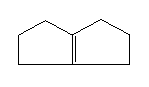will give:

(a)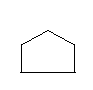(b)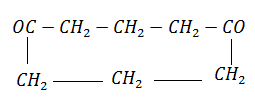(c)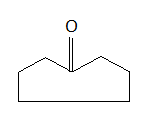(d) none of the above

Concept Questions :-

Alkanes, Alkenes and Alkynes - Chemical Properties
High Yielding Test Series + Question Bank - NEET 2020

Difficulty Level:

The product formed during the reaction,

$\mathrm{CH}\equiv \mathrm{CH}$ + NaOCl $\stackrel{0°\mathrm{C}}{\to }$ is:

(a) 1,2-dichloroethane                       (b) 1,2-dichloroethanal

(c) 1,2-dichloroethene                       (d) 1,2-dichloroethyne

Concept Questions :-

Alkanes, Alkenes and Alkynes - Chemical Properties
High Yielding Test Series + Question Bank - NEET 2020

Difficulty Level:

2-methylpropene is isomeric with butene-1. They can be distinguished by:

(a) Baeyer's reagent                         (b) ammoniacal ${\mathrm{AgNO}}_{3}$

(c) ${\mathrm{Br}}_{2}$ solution                                (d) ${\mathrm{O}}_{3}$$\mathrm{Zn}/{\mathrm{H}}_{2}\mathrm{O}$

Concept Questions :-

Alkanes, Alkenes and Alkynes - Chemical Properties
High Yielding Test Series + Question Bank - NEET 2020

Difficulty Level:

An octane number 100 is given to:

1. n-hexane                              2. iso-octane

3. neo-pentane                          4. neo-octane

Concept Questions :-

Alkanes, Alkenes and Alkynes -Methods of Preparation
High Yielding Test Series + Question Bank - NEET 2020

Difficulty Level:

Which of the following molecules/species are aromatic in character?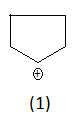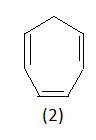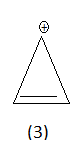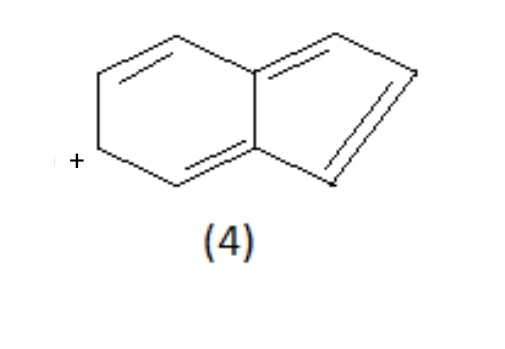(a) 2                                      (b) 3

(c) 4                                      (d) 1

Concept Questions :-

Aromatic Hydrocarbons - Huckel Rule of Aromaticity
High Yielding Test Series + Question Bank - NEET 2020

Difficulty Level:

Xylene on oxidation with acidic ${\mathrm{KMnO}}_{4}$ gives:

1. phthalic acid                          2. isophthalic acid

3. terephthalic acid                    4. all of these

Concept Questions :-

Aromatic Hydrocarbons - Benzene - Structure, Preparation & Chemical Reactions
High Yielding Test Series + Question Bank - NEET 2020

Difficulty Level:

Nitration of benzene is:

(a) nucleophilic substitution

(b) electrophilic substitution

Concept Questions :-

Aromatic Hydrocarbons - Benzene - Structure, Preparation & Chemical Reactions
High Yielding Test Series + Question Bank - NEET 2020

Difficulty Level:

Which of the following compounds has the lowest boiling point?

(a) ${\mathrm{CH}}_{3}{\mathrm{CH}}_{2}{\mathrm{CH}}_{2}{\mathrm{CH}}_{2}{\mathrm{CH}}_{3}$

(b) ${\mathrm{CH}}_{3}\mathrm{CH}=\mathrm{CH}-{\mathrm{CH}}_{2}{\mathrm{CH}}_{3}$

(c) ${\mathrm{CH}}_{3}\mathrm{CH}=\mathrm{CH}-\mathrm{CH}={\mathrm{CH}}_{2}$

(d) ${\mathrm{CH}}_{3}{\mathrm{CH}}_{2}{\mathrm{CH}}_{2}{\mathrm{CH}}_{3}$

Concept Questions :-

Aliphatic Hydrocarbon- physical properties
High Yielding Test Series + Question Bank - NEET 2020

Difficulty Level:

1-butyne on reaction with hot alkaline ${\mathrm{KMnO}}_{4}$ gives:

1. ${\mathrm{CH}}_{3}{\mathrm{CH}}_{2}{\mathrm{CH}}_{2}\mathrm{COOH}$

2. ${\mathrm{CH}}_{3}{\mathrm{CH}}_{2}\mathrm{COOH}$ + ${\mathrm{CO}}_{2}$

3. ${\mathrm{CH}}_{3}{\mathrm{CH}}_{2}\mathrm{COOH}$

4. ${\mathrm{CH}}_{3}{\mathrm{CH}}_{2}\mathrm{COOH}$ + HCOOH

Concept Questions :-

Alkanes, Alkenes and Alkynes - Chemical Properties